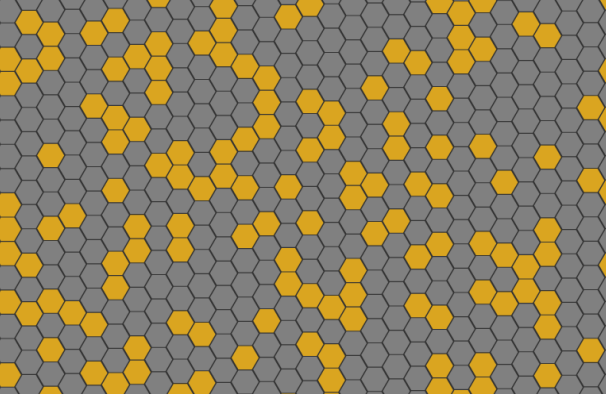# games-of-life

is a Mathematical solution to any Game of Life variation

## Demo

I created this hexagonal Game of Life demo to show that this package generalize the Game of Life in any of its variations. Click the image below to try it!## Installation

With npm do

``````npm install games-of-life
``````

You could also use a CDN adding this to your HTML page

``````<script src="https://unpkg.com/games-of-life/dist/games-of-life.min.js"></script>
``````

## Idea

On the 30th of May 2015, I have participated in a Coderetreat at Milan XPUG.

We have had so much fun coding implementations of the Game of Life.

As a mathematician, I think it is a very interesting problem. I couldn’t resist to generalize it and try to solve it in any of its variations.

A function getNeighboursOf, which returns the set of cells adjacent to a given cell, defines the shape of Game of Life universe.

Infact, since

``````getNeighboursOf(cell1) = getNeightboursOf(cell2) ⇒ cell1 = cell2
``````

it can be said that the set of neighbours of a cell is dual to the cell itself, hence the definition of the getNeighboursOf function is equivalent to the definition of the space of a Game of Life universe. Note that it defines the concept of nearness.

In other words,

if you define a getNeighbours function you also shape the space of a Game of Life universe

On the other hand, let be given the definition of an isAlive function, which returns `true` if the given cell is alive, `false` otherwise. It can be easily extended to an areAlive function which, given a list of cells, returns a list of booleans; following a similar identification we used for the getNeighboursOf function, an isAlive function describes the state of a Game of Life universe at a given moment.

The considerations above allow to implement an abstract Game of Life in a functional way, in any of its variations, for example:

• finite grid
• infinite grid
• 2-dimensional, 3-dimensional, n-dimensional
• square, triangular, hexagonal tiles
• cylinder, torus, moebius strip, boy surface

Take a look to createWorld.js for the implementation’s details.

The world has a transition rule which defaults to the classicTransitionRule.js.

## Example

A simple example is the infinite grid with two dimensional coordinates.

Define a getNeighboursOf which returns the neighbours of a given cell.

``````function getNeighboursOf (cell) {
var x = cell
var y = cell

var neighbours = []

for (var j = y - 1; j <= y + 1; j++) {
for (var i = x - 1; i <= x + 1; i++) {
if ((i === x) && (j === y)) {
continue
}

neighbours.push([i, j])
}
}

return neighbours
}

// Alias the adjacency function  with a more meaningful name,
// to improve semantic in the example code below.
var infiniteGrid2d = getNeighboursOf
``````

Create a Game of Life world, and get the evolve function

``````var gamesOfLife = require('games-of-life')

var createWorld    = gamesOfLife.createWorld
var transitionRule = gamesOfLife.classicTransitionRule.bind(null, 2, 3, 3)

var world = createWorld(infiniteGrid2d)

var evolve = world(transitionRule)
``````

The empty grid is represented by a function that always returns false, so

``````function emptyGrid () {
return false
}

evolve(emptyGrid) // will always return false
``````

Try with a single cell at the origin

``````function singleCellAtTheOrigin (cell) {
return ((cell === 0) && (cell === 0))
}

evolve(singleCellAtTheOrigin) // will always return false too, cause the cell dies
``````

Ok, a more interesting example is the blinker``````function horyzontalBlinker (cell) {
var x = cell
var y = cell

if (y !== 0) {
return false
}

if ((x >= -1) && (x <= 1)) {
return true
}

return false
}

var x = cell
var y = cell

if (x !== 0) {
return false
}

if ((y >= -1) && (y <= 1)) {
return true
}

return false
}
``````

You may check that the verticalBlinker evolves in the horyzontalBlinker and vice versa

``````for (var i = -1; i < 1; i++) {
for (var j = -1; j < 1; j++) {